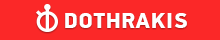GMAT Question of the Day - Daily to your Mailbox; hard ones only

 It is currently 26 Jun 2019, 11:19GMAT Club Daily Prep

Thank you for using the timer - this advanced tool can estimate your performance and suggest more practice questions. We have subscribed you to Daily Prep Questions via email.

Customized
for You

we will pick new questions that match your level based on your Timer History

Track

every week, we’ll send you an estimated GMAT score based on your performance

Practice
Pays

we will pick new questions that match your level based on your Timer History

Not interested in getting valuable practice questions and articles delivered to your email? No problem, unsubscribe here.Rectangles lie on sides AB, BC, and AC of ΔABC above. What is the sum

Author Message
TAGS:

Hide Tags

Math ExpertV
Joined: 02 Sep 2009
Posts: 55803
Rectangles lie on sides AB, BC, and AC of ΔABC above. What is the sum  [#permalink]

Show Tags

100:00

Difficulty:45% (medium)

Question Stats:44% (01:23) correct56% (00:50) wrongbased on 34 sessions

HideShow timer StatisticsRectangles lie on sides AB, BC, and AC of ΔABC above. What is the sum of the measures of the angles marked?

A. 90°
B. 180°
C. 270°
D. 360°
E. It cannot be determined from the information given

Attachment:2019-01-10_1338.png [ 19.89 KiB | Viewed 381 times ]

_________________
InternB
Joined: 10 Feb 2018
Posts: 33
GMAT 1: 550 Q46 V20Rectangles lie on sides AB, BC, and AC of ΔABC above. What is the sum  [#permalink]

Show Tags

The sum of exterior angles of a triangle is 360-Sum of angles of 3 rectangles ie 270
Would give us resulting measure of 90.

CEOP
Joined: 18 Aug 2017
Posts: 3947
Location: India
Concentration: Sustainability, Marketing
GPA: 4
WE: Marketing (Energy and Utilities)
Rectangles lie on sides AB, BC, and AC of ΔABC above. What is the sum  [#permalink]

Show Tags

Bunuel wrote:Rectangles lie on sides AB, BC, and AC of ΔABC above. What is the sum of the measures of the angles marked?

A. 90°
B. 180°
C. 270°
D. 360°
E. It cannot be determined from the information given

Attachment:
2019-01-10_1338.png

total sum of all three sides ; 360*3 = 1080
and for one side we have two sides of rectangle + 1 angle of triangle +1 external angle
2*90+angle a + ext a
total
3*2*90 + 180+ ext a+ext b+ext c = 1080
ext a+ext b+ext c= 360
IMO D
_________________
If you liked my solution then please give Kudos. Kudos encourage active discussions.

Originally posted by Archit3110 on 10 Jan 2019, 06:58.
Last edited by Archit3110 on 11 Jan 2019, 02:32, edited 2 times in total.
InternB
Joined: 15 Dec 2018
Posts: 30
Location: India
Schools: IIMA PGPX"20
GMAT 1: 650 Q45 V45GMAT 2: 650 Q45 V45GPA: 3.5
Re: Rectangles lie on sides AB, BC, and AC of ΔABC above. What is the sum  [#permalink]

Show Tags

IMO E

At first glance, you might finalize 180 but C and D vertices are differently aligned.

Posted from my mobile device
Math ExpertV
Joined: 02 Aug 2009
Posts: 7765
Re: Rectangles lie on sides AB, BC, and AC of ΔABC above. What is the sum  [#permalink]

Show Tags

1
1
Archit3110 wrote:
Bunuel wrote:Rectangles lie on sides AB, BC, and AC of ΔABC above. What is the sum of the measures of the angles marked?

A. 90°
B. 180°
C. 270°
D. 360°
E. It cannot be determined from the information given

Attachment:
2019-01-10_1338.png

chetan2u :
Hi could you please review the question and suggest the solution..

Hi..

Each vertice will make 360 when in a complete circle.
Now each vertice consists of two angles of rectangle, one angle at the exterior, say $$E_A$$and one angle of triangle, say A . So 2*90 +A+$$E_A$$

When we add all three vertices for the same .. we have 3*(2*90)+$$E_A+E_B+E_C$$+A+B+C..
We are looking for $$E_A+E_B+E_C$$ and A+B+C =180

So 3*(2*90)+$$E_A+E_B+E_C$$+180=3*360...540+180+$$E_A+E_B+E_C$$=1080...
$$E_A+E_B+E_C$$=1080-540-180=360

D
_________________
CEOP
Joined: 18 Aug 2017
Posts: 3947
Location: India
Concentration: Sustainability, Marketing
GPA: 4
WE: Marketing (Energy and Utilities)
Re: Rectangles lie on sides AB, BC, and AC of ΔABC above. What is the sum  [#permalink]

Show Tags

chetan2u

thank you

chetan2u wrote:
Archit3110 wrote:
Bunuel wrote:Rectangles lie on sides AB, BC, and AC of ΔABC above. What is the sum of the measures of the angles marked?

A. 90°
B. 180°
C. 270°
D. 360°
E. It cannot be determined from the information given

Attachment:
2019-01-10_1338.png

chetan2u :
Hi could you please review the question and suggest the solution..

Hi..

Each vertice will make 360 when in a complete circle.
Now each vertice consists of two angles of rectangle, one angle at the exterior, say $$E_A$$and one angle of triangle, say A . So 2*90 +A+$$E_A$$

When we add all three vertices for the same .. we have 3*(2*90)+$$E_A+E_B+E_C$$+A+B+C..
We are looking for $$E_A+E_B+E_C$$ and A+B+C =180

So 3*(2*90)+$$E_A+E_B+E_C$$+180=3*360...540+180+$$E_A+E_B+E_C$$=1080...
$$E_A+E_B+E_C$$=1080-540-180=360

D

_________________
If you liked my solution then please give Kudos. Kudos encourage active discussions.Re: Rectangles lie on sides AB, BC, and AC of ΔABC above. What is the sum   [#permalink] 11 Jan 2019, 02:26
Display posts from previous: Sort by

Rectangles lie on sides AB, BC, and AC of ΔABC above. What is the sum